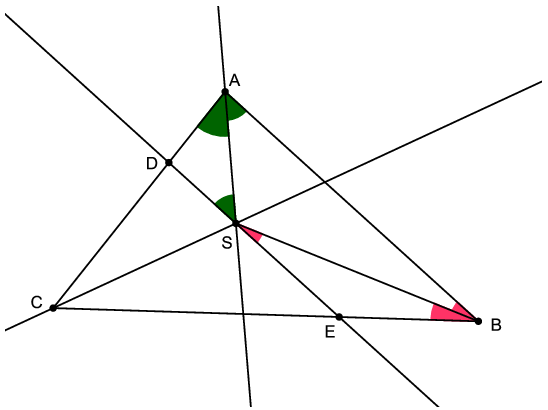Mathematical and Physical Journal
for High Schools
Issued by the MATFUND Foundation
 Already signed up? New to KöMaL?

#Problem K. 384. (September 2013)

K. 384. Triangle ABC has an obtuse angle at vertex A. Denote the centre of the inscribed circle by S. The line drawn through S parallel to AB intersects side AC at D and side BC at E. Prove that DE=AD+BE.

German competition problem

(6 pont)

Deadline expired on October 10, 2013.

Sorry, the solution is available only in Hungarian. Google translation

Megoldás. $\displaystyle BAS\angle=DSA\angle$, mert váltószögek. $\displaystyle DAS\angle=BAS\angle$, mert $\displaystyle AS$ szögfelező. Így $\displaystyle DSA\angle=DAS\angle$, vagyis az $\displaystyle ADS$ háromszög egyenlő szárú. Így $\displaystyle AD=DS$.

Hasonlóan lehet belátni, hogy $\displaystyle BE=SE$. Így pedig $\displaystyle DE=DS+SE=AD+BE$.### Statistics:

 202 students sent a solution. 6 points: 160 students. 5 points: 18 students. 4 points: 4 students. 3 points: 8 students. 2 points: 1 student. 0 point: 9 students. Unfair, not evaluated: 2 solutionss.

Problems in Mathematics of KöMaL, September 2013